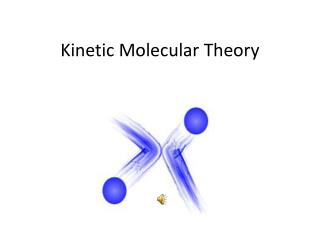DownloadDownload PresentationKinetic Molecular Theory

# Kinetic Molecular Theory

Download Presentation## Kinetic Molecular Theory

- - - - - - - - - - - - - - - - - - - - - - - - - - - E N D - - - - - - - - - - - - - - - - - - - - - - - - - - -
##### Presentation Transcript

1. Kinetic Molecular Theory

2. Kinetic Molecular Theory 1. Gases consist of large numbers of molecules in continuous, random motion. 2. The volume of the molecules of a gas is negligible compared to the total volume in which the gas is contained. 3. Attractive and repulsive forces between gas particles are negligible. 4. Energy can be transferred between molecules during collisions but the average kinetic energy remains the same for a given temperature. 5. The average kinetic energy of the molecules is proportional to absolute temperature.

3. KEavg = 3/2RT rms = 3RT/M R = 8.31 J/mol.K M = mass of a mole of gas in kg rms = root mean square velocity

4. diffusion = mixing of gases effusion = passage of gas through a hole or membrane Graham's Law of Effusion: rate1/rate2 = √(molar mass2/molar mass1) effusion diffusion

5. Real Gases exhibit near ideal behavior at low pressure and high temperature because the molecules interact less under these conditions and the volume of the particles is less important compared to the total volume. ideal PV = nRT real P = nRT/(V-nb) -a(n/V)2 Pressure correction for intermolecular attractions Volume correction for nonzero particle volume